Propellant-less Electromagnetic Propulsion

By

Stavros G. Dimitriou
TEI-Athens, Dept. of Electronics Engineering
E-mail: dimsta@ee.teiath.gr

And

Dr. David King
The University of Manchester
Division of Electrical Engineering
E-mail: david.king@man.ac.uk

Abstract :

It is shown that the electric current and its rate of change can emulate the physical velocity and acceleration respectively. This emulation can be practically implemented, by means of electronic circuits which demonstrate the physical validity of the theory These electronic circuits are coupled to specially shaped "antennas" which induce the relative states of velocity and/or acceleration to the region of space adjacent to them.
The relative state of velocity can be used to emulate the condition of subjecting a mass to a centrifugal force relative to the center of the Earth and lifting it. The relative state of acceleration can be used to induce force and thus thrust on a target mass.
The relevant circuits are compact, easily implemented and allow the control of the intensity and the direction of the resulting thrust force. Their "antenna" typically has the form of a disk or rod, electrically behaving as a resonant tank circuit. or plane capacitor, depending on case.

1. INTRODUCTION
We summarize below some concepts pertaining to propulsion and their evolution into an ongoing, expanded research topic. This work derives relationships linking the electric charge and its time-derivatives with space and its time-derivatives too. Implementation ideas are also presented, as well as techniques to achieve control of the generated propulsion by electrical means.

2. DERIVATION OF THE BASIC RELATIONSHIPS
The derivation of the basic relationships can be found in  The coupling formulae thus derived are the following, assuming changes with time are sufficiently slow as to permit considering for any practical purpose that the component of the electric field is the dominant one: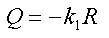(1)

Taking the first and second derivative of eq.(1) with respect to time yields :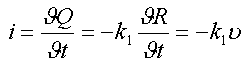(2)

and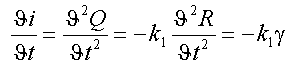(3)

whereand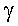represent electric current, velocity and acceleration, respectively.

Equations (2) and (3) hint at the possibility of emulating and/or substituting a physical velocity or acceleration by an electric current and its derivative with respect to time.
The relevant vectors are coincident with the orientation of the line extending from the source to the observer. while heir direction depends on the sign of the corresponding derivative.

3. VELOCITY AND ACCELERATION FROM A PLANE CAPACITOR
As reasoned in , similar equations can be derived for the case of a plane capacitor: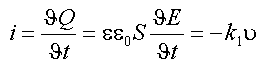(5)

and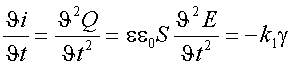(6)

Where E and S stand for the intensity of the electric field and the area of the plane capacitor, respectively.
Equations (5) and (6) suggest the possibility of generating a field of velocity (simulating an inertial frame of reference) and/or a field of acceleration by means of the electric field in a plane capacitor . Rod-shaped structures are also feasible..

4. HOVERING AT ALMOST NO ENERGY INPUT
The creation of a net lift force can be shown to be analogous to developing a centrifugal lift on a body moving normal to the terrestrial vector of gravity. A major advantage however is that in using equivalent states of velocity, induced electromagnetically, no physical movement of the body tangential to the surface of the
Earth is needed. A further asset is that in inducing electromagnetically the state of equivalent velocity, the actual lifting energy is of relativistic origin and is supplied by conversion of a tiny portion of the mass to be lifted into equivalent potential energy. In this case, the electromagnetic induction of the state or domain of equivalent acceleration simply catalyses and sustains this energy conversion, as it will be shown in the current Ph.D. work The result is a very high efficiency in upward thrust generation. As no input energy is demanded, the lifted body is isolated in its own frame of reference and is therefore no subject to inertia.

It can be shown that the gain in height obtained by such a system is expressed by: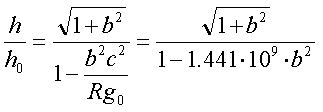(4)

where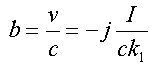, and I is the current on the radiating structure R is the mean Earth radius and go is the gravitational acceleration on the surface of the Earth.
The whole process is best attained in high-Q electromagnetic resonators, such as specially shaped transmission lines and cavities, with dimension from a fraction of the metre to several metres across. The resonance frequencies involved range from VHF down to HF respectively. It can be shown that the efficiency of conversion grows with the volume of the relevant structure and depends on the particular shape of the structure. Pan-cake or convex-lens shaped cavities and transmission lines are strong candidates in this application, aided by the utilization of almost conventional RF power techniques .

5. WAVEFORMS USED
The electric waveforms used to generate the vectors of velocity and/or acceleration must have dissimilar slopes between the ascending and descending part of the signal. This is necessary in order to obtain a non-zero sum of the derivatives per period. Extensive analysis has been carried in  to optimize the parameters pertaining to each particular waveform.
The efficiency of the electrically generating domains of velocity and acceleration depends on the dimensions of the generating element, with regard to the fundamental wavelength of the waveform applied to it, as stated above.

6. MODULATED WAVEFORMS
In order to increase the efficiency of the radiating structure, which is very low at relatively low frequencies, waveforms with varying rise- and fall- slopes due to progressively varying amplitude or frequency can be used.
The inherent advantage of the modulated waveforms is that almost conventional techniques can be used to generate thrust from appropriate radiating structures. A second advantage is that an appropriately modulated RF carrier of a sufficiently high frequency can be easily beamed and/or focused to a desirable direction and object, in order to cause thrust generation on it.
The use of tuned parts of transmission lines and specially shaped cavity resonators is implicitly imperative in terms of the Q benefits it can provide. More advanced forms of modulation are currently into investigation.
The sign of the acceleration can be controlled by the duty cycle of the modulating waveform, which can also provide a neutral position. Throttling action can thus be achieved, apart from the possibility of controlling the amplitude through the power supply. More advanced forms of carrier modulation are presently researched on, providing a much higher yield.

7. IMPLICATIONS AND APPLICATIONS
The exploitation of the creation of states of equivalent acceleration due to the second derivative of the electric charge with respect to time can lead to the production of thrust vectors of arbitrarily controlled orientation and amplitude. These vectors can be used for space station keeping as a first application, and as a substitute to more conventional thrusters at a later stage of development. Thrusters in the form of RF- resonant disks, rod-like transmission lines and plane-capacitor banks are currently researched. Microwave stripline IC's for thrust generation should also be possible to form.

8. ACKNOWLEDGEMENT
This work was initiated as an M.Sc./Ph.D. research at the University of Manchester, supervised by Dr. David King . Following its broadened interest  it is presently continued as a joint research with the added cooperation of the Department of Physics-Chemistry and Materials Technology (Prof.'s. N.Patarghias, G. Kalkanis and D. Vattis) and the Department of Electronics Engineering of TEI-Athens, in Greece.

References:
 S. G. Dimitriou, Radiation Phenomena of Specially Shaped Current Pulses, M.Sc. Thesis, The University of Manchester, (1994).

 S. G. Dimitriou, Propulsive effect on a massive plane capacitor driven by slope - asymmetric pulses, URSI International Symposium On Electromagnetic Theory, 25-28 May 1998, pp. 805-7,Thessaloniki, Greece.

 S. G. Dimitriou, On the pendulum oscillations of a suspended RF resonant circuit, First antigravity conference, Reno, Nevada, June 27-28, 1999.

 Thrust from time-derivatives of the electric charge, by S. G .Dimitriou, a paper presented at the Greenglow project conference at the University of Lancaster, on Sept. 3 1998, sponsored by this University and British Aerospace.

This paper has been published on this web site, courtesy of Dimitriou Stavros